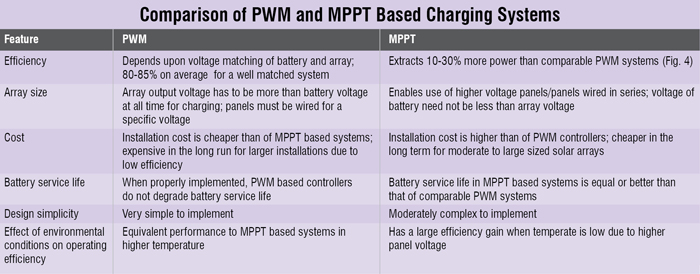Wednesday, September 27, 2023

# Efficiently Harnessing Solar Power With Tracking System

Solar power holds a lot of promise for countries like India that are blessed with ample sunshine most of the time. But making solar power economically viable is a challenge that many are grappling with still. Here are some suggestions -- Sarang Gupta

India has enormous potential for solar power, as it receives 5000 trillion kWh worth of energy from the sun every year. The Jawaharlal Nehru National Solar Mission aims to promote solar power in India and help India achieve self-sufficiency in energy. Though the cost of solar power (Fig. 1) has been falling steadily, it is still more expensive than existing solutions. Hence it fulfils only a minor fraction of our ever-growing energy needs.

It is therefore imperative that, to increase production and decrease the cost per kilo watt hour, the currently installed solar power be used efficiently. This goal can be achieved with the help of maximum power point tracking (MPPT).

An MPPT system extracts maximum power output from a given solar cell. The power output of a solar cell is nonlinear and dependent on solar irradiation or intensity, ambient temperature and the load voltage. This power output can be seen with the help of a current-voltage (I-V) (Fig. 2) graph. Since power output is the product of current and voltage, it is signified by the area of the rectangle formed by a given point on the I-V curve and the origin. After finding voltage at which power output is highest, the MPPT system applies an appropriate load resistance to the solar panel, thus maximising the power output. Usually, the MPPT then uses a voltage converter (DC-DC/DC-AC) to output a voltage appropriate for the given load or to charge batteries.

Algorithms used in MPPT systems
MPPT can be implemented by a system in several ways. Each MPPT algorithm has its own advantages and drawbacks, and is hence suited for different conditions like:

Hill Climb or Perturb and Observe. Perturb and Observe (P&O) is the most popular MPPT method due to its effectiveness and simplicity. It uses the characteristic I-V curve of a solar panel to determine the point of maximum power. The MPPT system increases the voltage of the panel such that the power obtained increases until there is no change in power. Since the power increases in the direction of voltage until a ‘knee point’ or a ‘cliff’ in the graph, this method is also called the Hill Climb method. This method may, though, produce oscillations in the output due to dynamic changes in voltage.

Incremental Conductance. Another popular method of maximum power point tracking is Incremental Conductance (IC) method. In IC method, MPPT controller uses the incremental conductance (given by dI/dV, which is approximately equal to ΔI/ΔV for small changes in voltage and current) to find the voltage at which maximum power is delivered. This voltage is reached when the incremental conductance is equal to the conductance of the array. The process is repeated when the irradiance changes. A drawback of the IC method is that, the voltage oscillates due to the readjustment of MPPT voltage.

Constant Voltage. Constant Voltage method is the simplest of all methods but its simplicity comes at a cost to efficiency. The MPPT system interrupts load to the solar panel and measures the voltage known as the open circuit voltage (Voc). The ratio of the MPPT voltage and the Voc is a constant k (k<1). The value of k is a characteristic of the given panel and is determined either by modelling the solar panel system and operating conditions or by experimentation. It is approximately equal to 0.76 for most panels. This method is extremely easy and cheap to implement as it can be implemented without a microcontroller. The value of k is static for the system and results in an approximation of the actual MPPT voltage.Constant Current. Constant Current method is similar to the Constant Voltage method but is slightly more complicated due to addition of a switch and a load to measure the Isc (short-circuit current). This also results in heat dissipation in the MPPT module. The constant k is taken as the ratio of MPPT current and Isc. The method suffers from the same drawbacks as those faced by the Constant Voltage method.